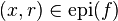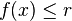# Epigraph (mathematics) facts for kids

Kids Encyclopedia Facts

In mathematics, the epigraph of a function is the set of paired values so the output of the function at the first value is less than or equal to the second value. In math terms this means$(x,r) \in \operatorname{epi}(f)$ if and only if$f(x) \leq r$.Epigraph (mathematics) Facts for Kids. Kiddle Encyclopedia.